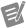From 1:00 AM - 02:00 AM CST on Saturday, July 4, ni.com will be undergoing system upgrades that may result in temporary service interruption.

We appreciate your patience as we improve our online experience.

# Bridge Scaling Types

NI-DAQmx provides three methods to scale electrical values (voltage ratios) from a bridge-based sensor to physical units:

• Two-Point Linear—You provide two pairs of electrical values and their corresponding physical values. NI-DAQmx uses those values to calculate the slope and y-intercept of a linear equation and uses that equation to scale electrical values to physical values. Measured electrical and physical values can fall outside the range of the values specified for calculating the slope and y-intercept.
• Table—You provide a set of electrical values and the corresponding physical values. NI-DAQmx performs linear scaling between each pair of electrical and physical values. The input limits must fall within the smallest and largest physical values.
• Polynomial—You provide the forward and reverse coefficients of a polynomial equation. NI-DAQmx uses that equation to scale electrical values to physical values. Use the DAQmx Compute Reverse Polynomial Coefficients VI/function to determine one set of coefficients if you know only the other set.

Datasheets or calibration certificates from sensor manufacturers often include a table of electrical and physical values or a polynomial equation for scaling. If you do not have a table or polynomial equation for your sensor, use two-point linear scaling. Use the rated output of the sensor and the sensor capacity as one pair of electrical and physical values. Use zero for the other pair of electrical and physical values.Note

Strain gages use specific equations for scaling, depending on the bridge configuration.